Get instant live expert help with Excel or Google Sheets“My Excelchat expert helped me in less than 20 minutes, saving me what would have been 5 hours of work!”

#### Post your problem and you’ll get expert help in seconds.

Your message must be at least 40 characters
Our professional experts are available now. Your privacy is guaranteed.

# Learn How to Use INDEX and MATCH on Multiple Columns in Excel

We can use an array formula that is based on the MMULT, TRANSPOSE, COLUMN, and INDEX functions to lookup a value by matching across multiple columns. The steps below will walk through the process.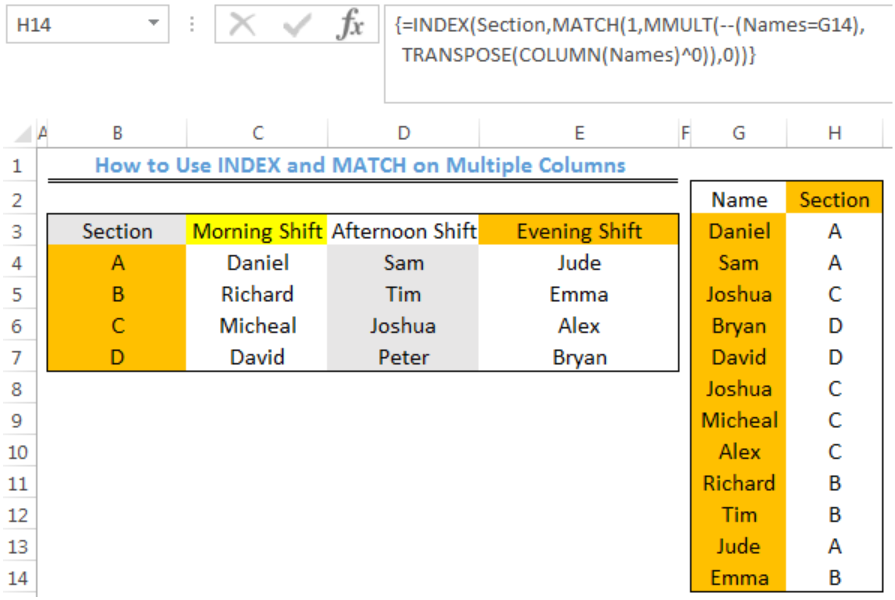Figure 1- How to Use INDEX and MATCH functions on Multiple Columns

## General Formula

`=INDEX(range1,MATCH(1,MMULT(--(range2=criteria),TRANSPOSE(COLUMN(range2)^0)),0))`

## Formula

`=INDEX(Section,MATCH(1,MMULT(--(Names=G3),TRANSPOSE(COLUMN(Names)^0)),0))`

## Setting up the Data

We will use the INDEX and MATCH functions to get the Sections where different workers are stationed as shown in figure 2.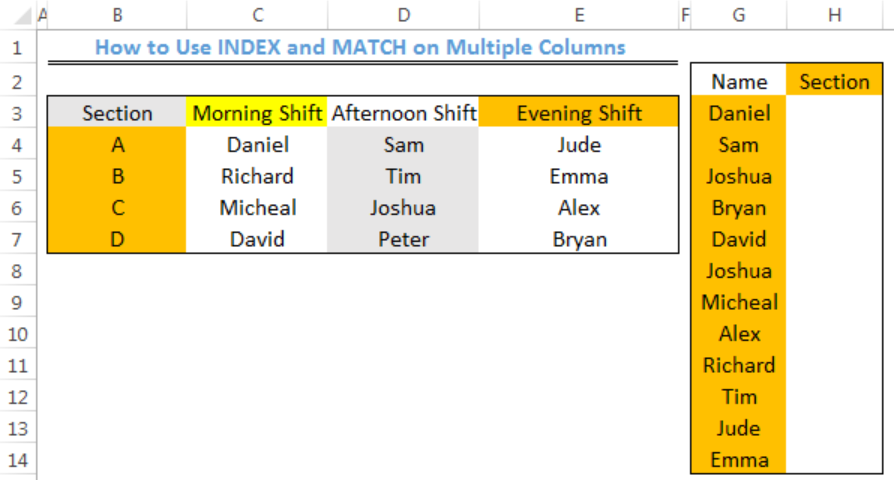Figure 2 – Setting up the Data

Note: Cell B4 to Cell B7 is a named range called “Sections.” To name this range, we will select Cell B4 to Cell B7. We will click on B4 in figure 3. We will enter the name of the range as “Section” and press enter. We will do a similar thing for the named range, C4:E7 as “Names”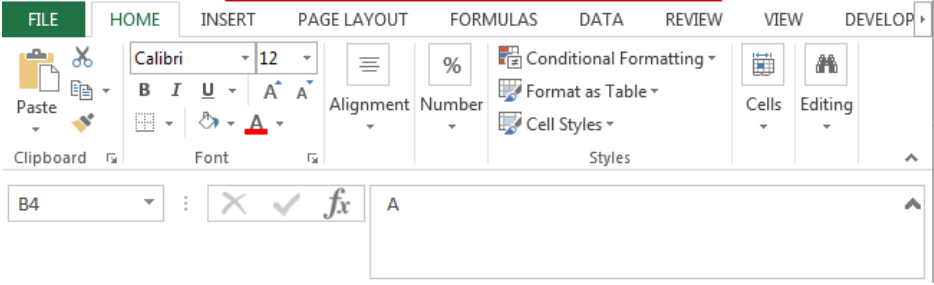Figure 3 – Naming the Ranges

## Lookup Names with INDEX and MATCH on Multiple Columns

• We will click on Cell H3
• We will insert the formula below into Cell H3
`=INDEX(Section,MATCH(1,MMULT(--(Names=G3),TRANSPOSE(COLUMN(Names)^0)),0))`
• Because this is an array formula, we will press CTRL+SHIFT+ENTER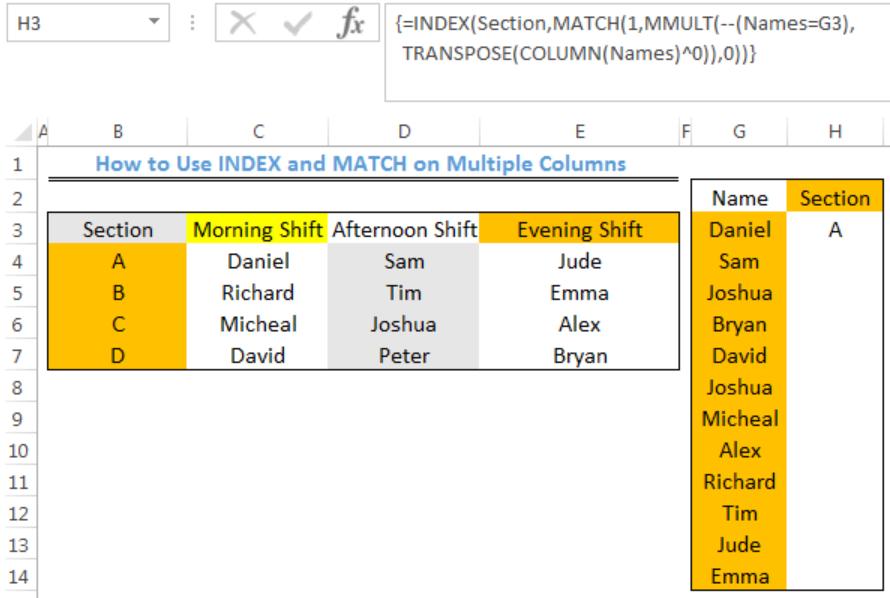Figure 4- Lookup Names with INDEX and MATCH functions on Multiple Columns

• We will click on Cell H3 again
• We will double click on the fill handle tool which is the small plus sign you see at the bottom right of Cell H3. Select and drag down to copy the formula to Cell H14.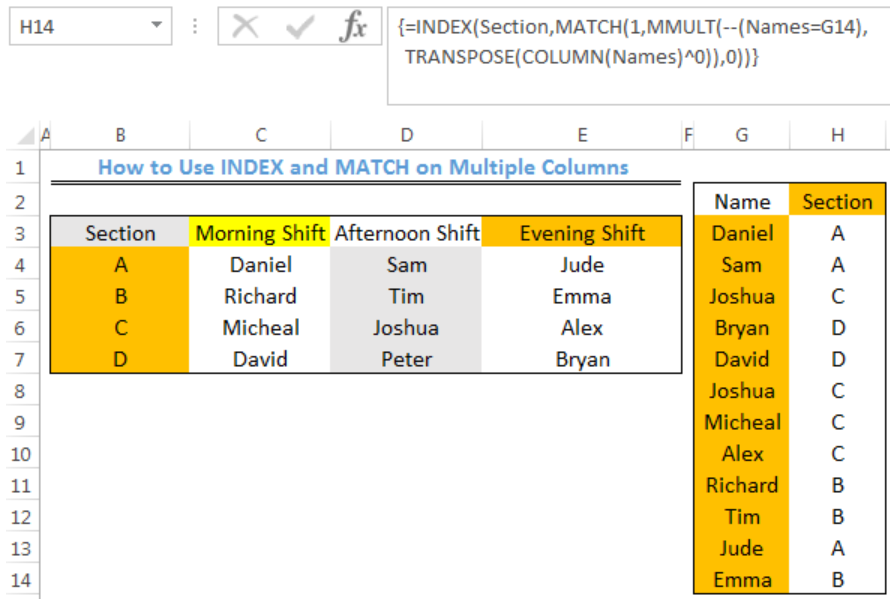Figure 5- Result of Lookup Names with INDEX and MATCH functions on Multiple Columns

## Explanation

The criteria in this formula is: –(names=G3). This criteria results in the generation of TRUE or FALSE results for the values in the data. The double negative forces the TRUE and FALSE values to produce 1 and 0 in an array like this:

`{1,0,0;0,0,0;0,0,0;0,0,0}`

This array is 4 rows by 3 columns, matching the structure of “Names”.

The TRANSPOSE section of the formula creates a second array in this form: TRANSPOSE(COLUMN(Names)^0))

The COLUMN function creates a numeric array having 3 columns and 1 row. The TRANSPOSE FUNCTION converts this array to 1 column and 3 rows. Raising to the power of zero simply converts all numbers in the array to 1. The MMULT function then performs matrix multiplication:

`MMULT({1,0,0;0,0,0;0,0,0;0,0,0},{1;1;1})`

The MATCH function receives the result from the MMULT FUNCTION. The lookup value of MATCH is 1.

`MATCH(1,{1;0;0;0},0)`

The MATCH function returns the position of the first match, which corresponds to the row of the first matching row meeting the supplied criteria. This is feed into INDEX as the row number, with the named range “Section” as the array:

`=INDEX(Section,1)`

Finally, INDEX returns “A.”

## Instant Connection to an Expert through our Excelchat Service

Most of the time, the problem you will need to solve will be more complex than a simple application of a formula or function. If you want to save hours of research and frustration, try our live Excelchat service! Our Excel Experts are available 24/7 to answer any Excel question you may have. We guarantee a connection within 30 seconds and a customized solution within 20 minutes.

Solution examplesI need a formula to compare the data in two columns and then export the mismatched data in the 3rd column
Solved by S. Q. in 20 minsI need to sum the number of hours per month one of three halls are being rented
Solved by A. F. in 60 minsUse the Vlookup Function to complete the "employee" column of table 2. Use "job Id" from table 2 as your lookup_value(s) and table 1 as your reference.
Solved by C. H. in 16 minsIf a cell in another sheet is populated I need a vlookup done. If the cell is not populated I need the cell to return blank.
Solved by T. D. in 60 minsI am trying to make a chart that turns a week range red if nothing is entered in the range. If something is entered then I would like it to turn green. Please Help
Solved by E. U. in 43 mins